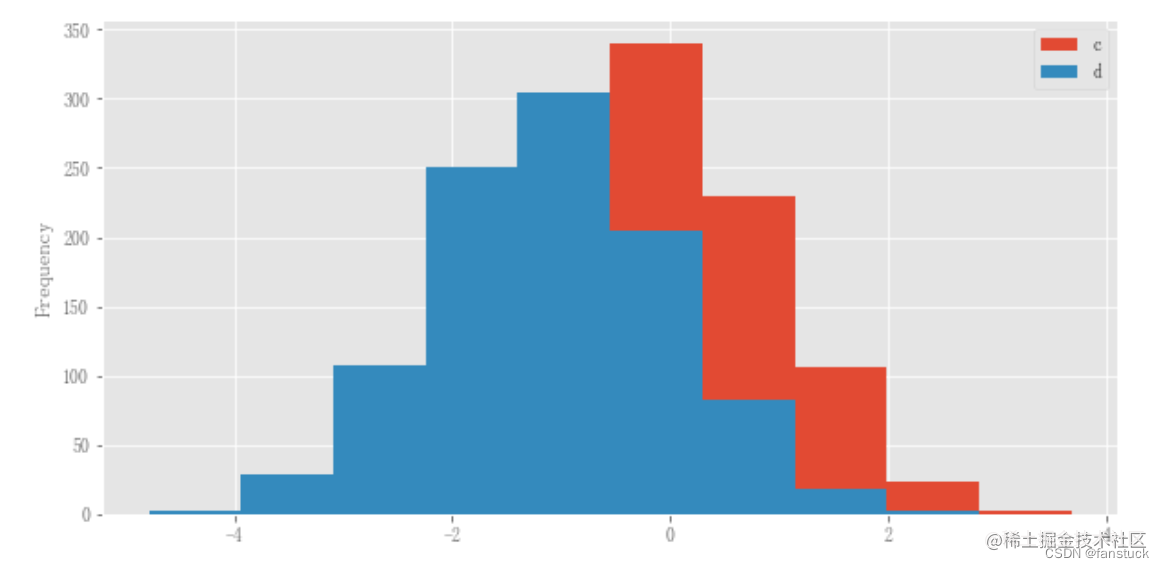# 前言

Pandas数据分析系列专栏已经更新了很久了，基本覆盖到使用pandas处理日常业务以及常规的数据分析方方面面的问题。从基础的数据结构逐步入门到处理各类数据以及专业的pandas常用函数讲解都花费了大量时间和心思创作，如果大家有需要从事数据分析或者大数据开发的朋友推荐订阅专栏，将在第一时间学习到Pandas数据分析最实用常用的知识。此篇博客篇幅较长，涉及到处理文本数据(str/object)等各类操作，值得细读实践一番，我会将Pandas的精华部分挑出细讲实践。博主会长期维护博文，有错误或者疑惑可以在评论区指出，感谢大家的支持。

# 一、基础绘图：plot

Series和DataFrame上的plot方法只是plt.plot（）的简单包装,这里我们用一段实际数据来进行可视化展示：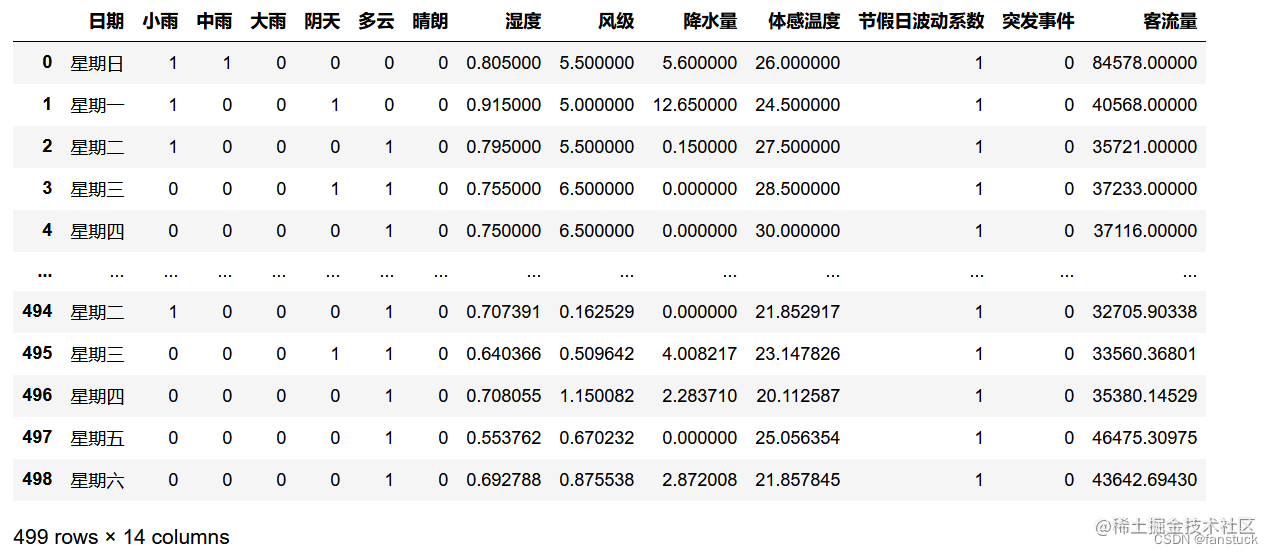`df_flow['客流量'].plot()`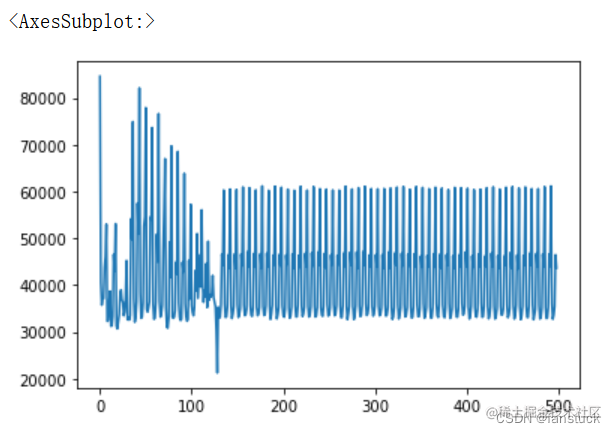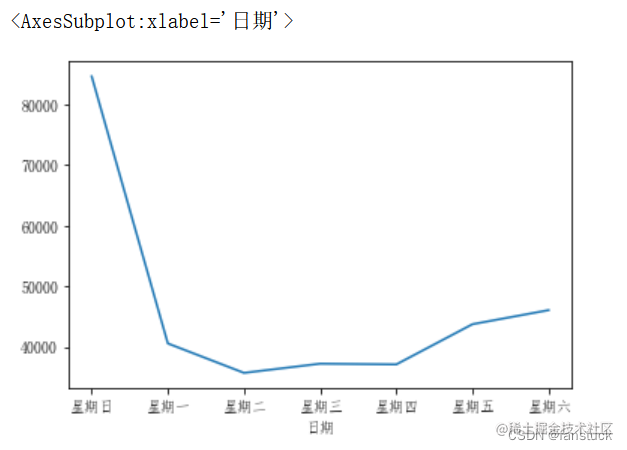`df_flow_mark[['湿度','风级','降水量']].plot()`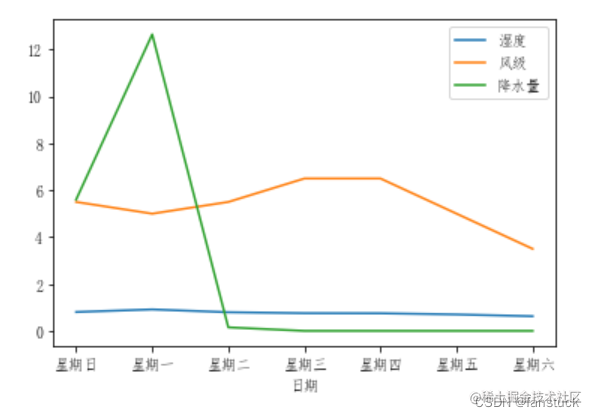```df_flow_7=df_flow[df_flow['日期']=='星期日'].iloc[:7,:] df_flow_7.rename(columns={'客流量':'星期日客流量'},inplace=True) df_flow_6=df_flow[df_flow['日期']=='星期六'].iloc[:7,:] df_flow_6.rename(columns={'客流量':'星期六客流量'},inplace=True) df_compare=pd.concat([columns_convert_df(df_flow_7['星期日客流量']),columns_convert_df(df_flow_6['星期六客流量'])],axis=1) df_compare.plot(x='星期日客流量',y='星期六客流量')```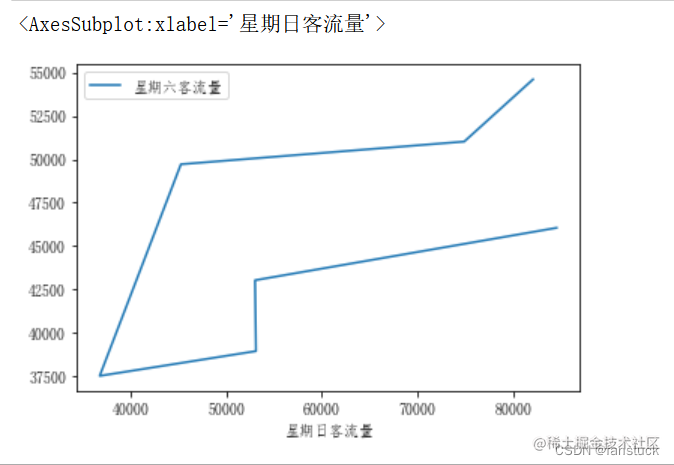# 二、底图板块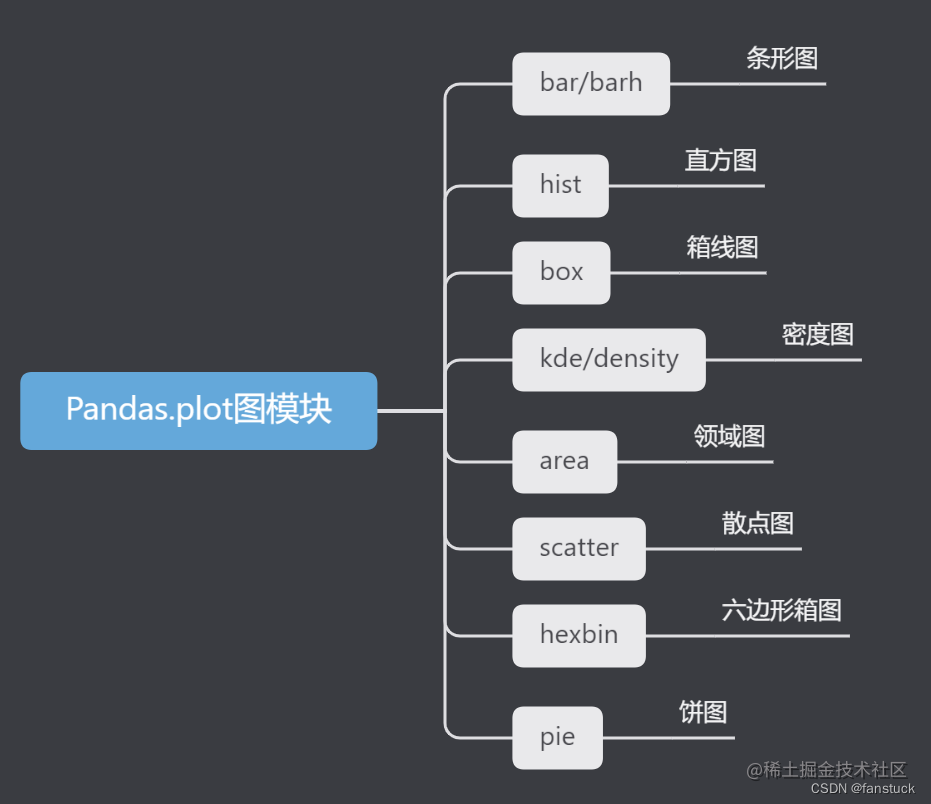``` df.plot.area df.plot.barh df.plot.density df.plot.hist df.plot.line df.plot.scatter df.plot.bar df.plot.box df.plot.hexbin df.plot.kde df.plot.pie

``` 除了这些类型，还有DataFrame.hist（）和DataFrame.boxplot（）方法，它们使用单独的接口。

## 1.条形图

`df_flow_mark['客流量'].plot(kind='bar')`

`df_flow_mark['客流量'].plot.bar()`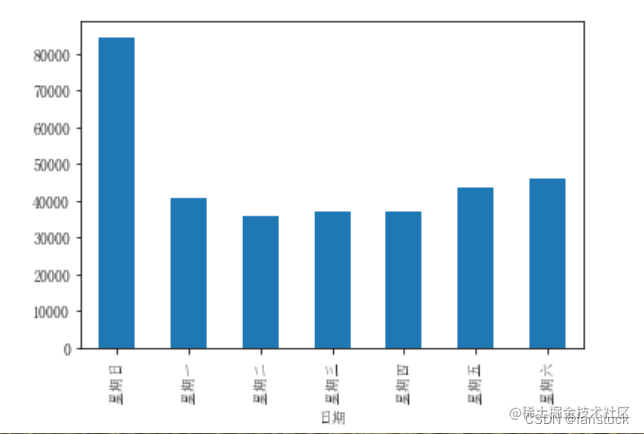`df_flow_mark[['风级','降水量']].plot.bar()`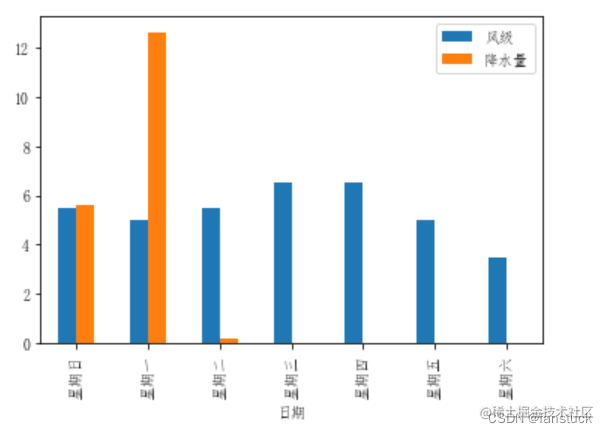`df_flow_mark[['风级','降水量']].plot.bar(stacked=True)`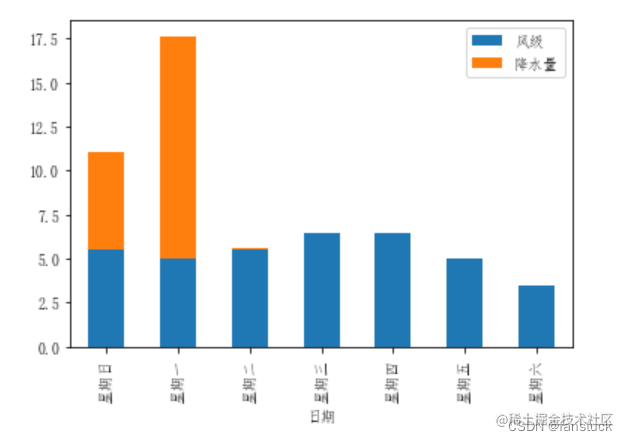`df_flow_mark[['风级','降水量']].plot.barh(stacked=True)`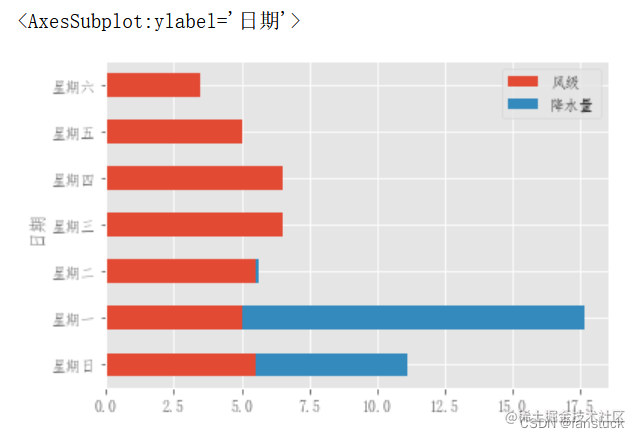## 2.直方图

```df4 = pd.DataFrame( { "a": np.random.randn(1000) + 1, "b": np.random.randn(1000), "c": np.random.randn(1000) - 1, }, columns=["a", "b", "c"], ) plt.figure(); df4.plot.hist(alpha=0.5)```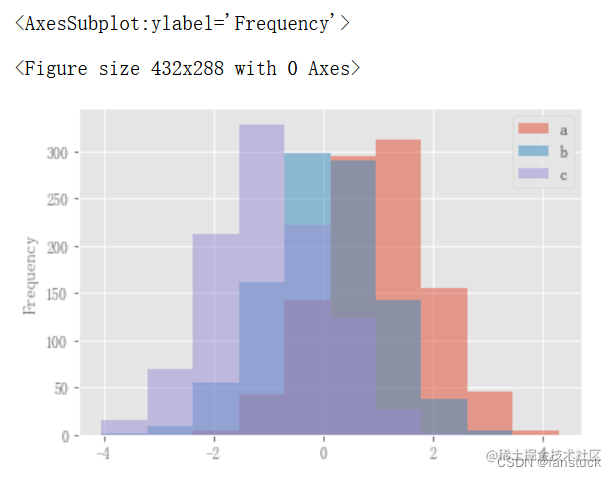直方图可以使用stacked=True进行叠加。可以使用bins关键字更改bin大小。

`df4.plot.hist(stacked=True, bins=20);` 可以传递matplotlib hist支持的其他关键字。例如，水平和累积直方图可以通过`orientation='horizontal'``cumulative=True`绘制。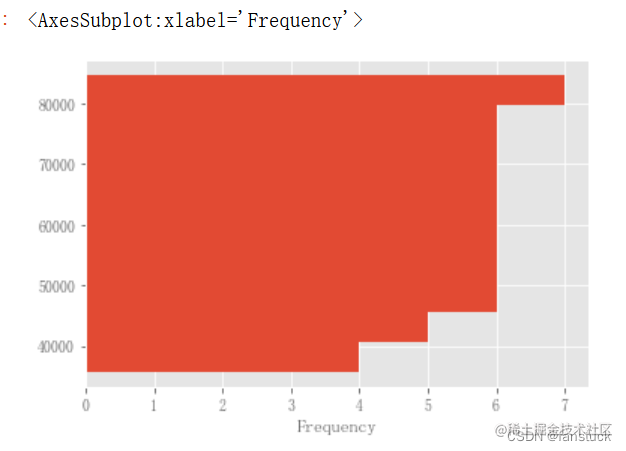```plt.figure(); df_flow_mark['风级'].hist();```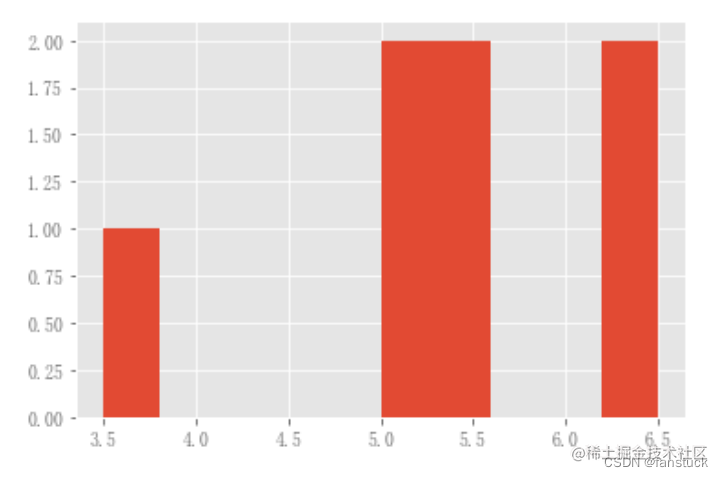DataFrame.hist()可以在多个子地块上绘制列的直方图：

```plt.figure(); df_flow_mark[['风级','降水量']].diff().hist(color="k", alpha=0.5, bins=50);```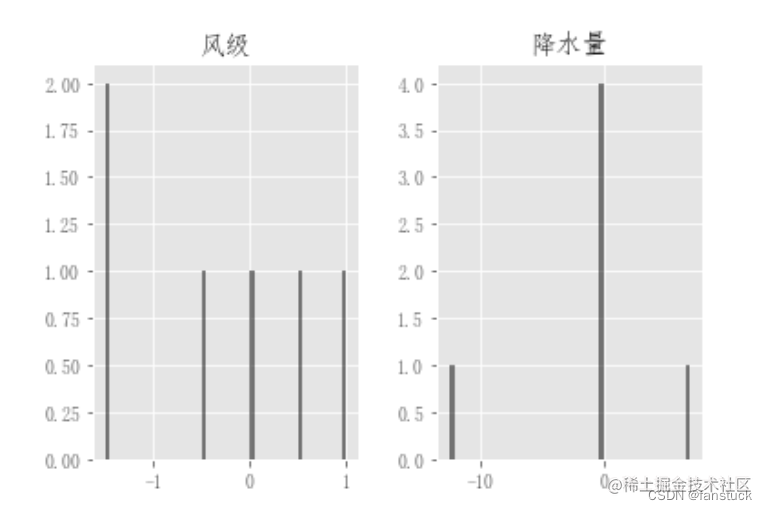``` data = pd.Series(np.random.randn(1000))

data.hist(by=np.random.randint(0, 4, 1000), figsize=(6, 4)); ```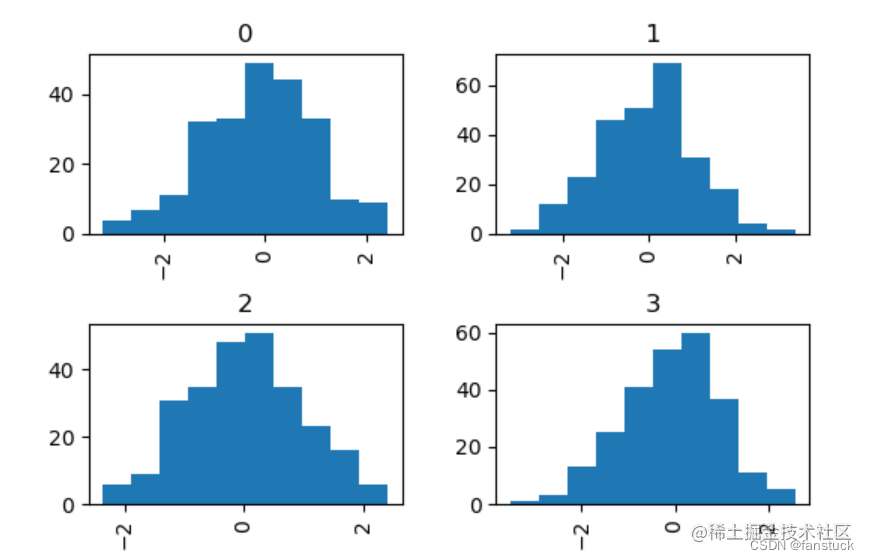```data = pd.DataFrame( { "a": np.random.choice(["x", "y", "z"], 1000), "b": np.random.choice(["e", "f", "g"], 1000), "c": np.random.randn(1000), "d": np.random.randn(1000) - 1 } ) data.plot.hist(by=["a", "b"], figsize=(10, 5));```